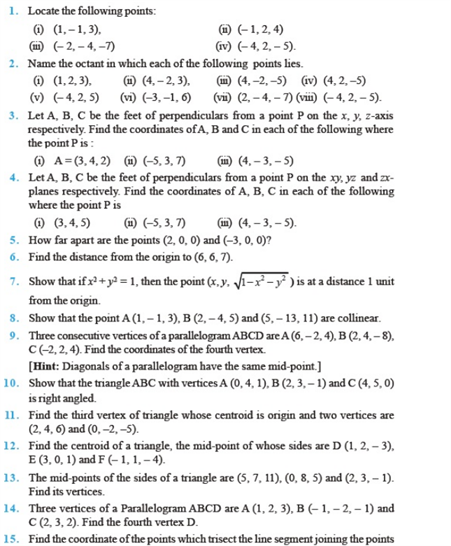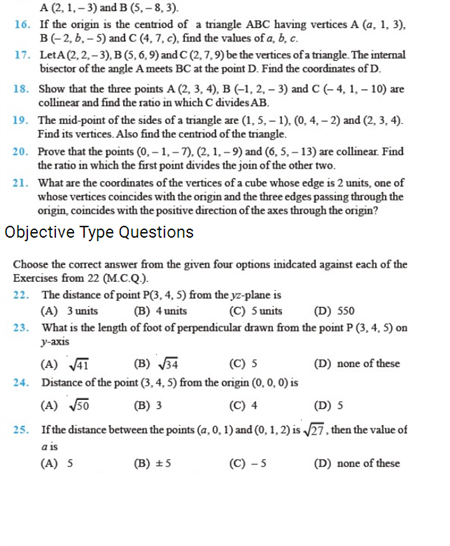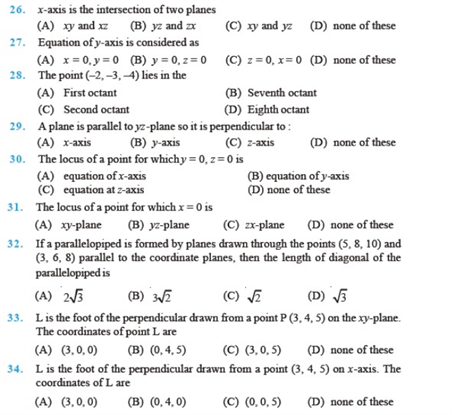# Chapter 12 - Introduction to 3-D Geometry

Introduction to 3-dimensional geometry class 11 chapter 12 is one of the most important topics to understand. You will find important questions on this topic to prepare well for class 11 exams. Practising questions on (CBSE) will help you understand the concept of 3- dimensional space.

Also, check,

• Three-Dimensional Geometry for Class 12

Chapter 12 of class 11 shall be studied thoroughly by practicing extra questions on this topic as it is also repeated in class 12. Three-dimensional geometry is an important topic for class 12 CBSE board exams.

## Important Questions of Introduction to 3-D Geometry Class 11 (Chapter  12)

Question 1: Find the coordinates of a point equidistant from the four points O (0,0,0), A(a, 0, 0), B(0, b, 0) and C(0,0, c). (Solution: (a/2, b/2, c/2)).

Question 2: Find the distance between the points P(-2,4,1) and Q(1, 2, – 5). (Solution: 7 units).

Question 3: Find the locus of the point which is equidistant from the points A(0,2,3) and  (2, -2, 1). (Solution: x – 2y – z +1 = 0).

Question 4: Prove that the points (a,b,c), (b,c,a) and (c,a,b) are the vertices of an equilateral triangle.

Question 5: Find the ratio in which the line joining (2,4,5) and (3,5,4) is divided by the yz-plane. (Solution: 2:3 externally)

Question 6: Write the distance of point P(2,3,5) from the xy-plane. (Solution: 5)

Question 7: If the origin is the centroid of a triangle ABC having vertices A(a,1,3), B(-2,b,-5) and C(4,7,c), find the values of a,b,c. (Solution: -6,5,-8).

Question 8: Show that the lines joining the vertices of a tetrahedron to the centroids of the opposite faces are concurrent.

Question 9: The midterms of the sides of a triangle are (1,5,-1), (0,4,-2) and (2,3,4). Find its vertices. (Solution: A (1,2,3), b(3,4,5), c(-1, 6, -7))

Question 10: Name the octants in which the following points lie:

(-5,-4,7), (-7,2,-5) (Solutions – X’OY’Z , X’OYZ’)

### NCERT Questions On Chapter 12 Class 11 – Introduction to the Three Dimensional Coordinate Geometry